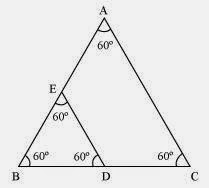# ABC and BDE are two equilateral triangles such that D is the mid-point of BC. Ratio of the area of triangles ABC and BDE is (A) 2 : 1 (B) 1 : 2 (C) 4 : 1 (D) 1 : 4

ΔABC and ΔBDE are two equilateral triangle. D is the midpoint of BC.BD = DC = 1/2BC

Let each side of triangle be 2a.

As, ΔABC ~ ΔBDE

∴ Area(ΔABC)/Area(ΔBDE) = AB2/BD2 = (2a)2/(a)2 = 4a2/a2 = 4/1 = 4:1(23)(1)